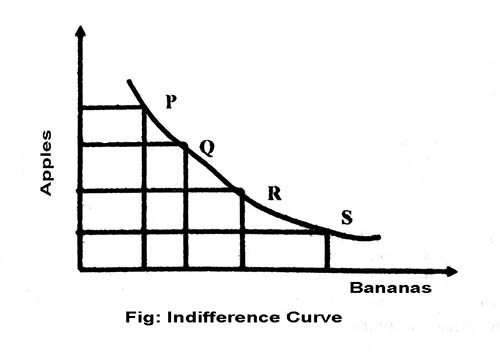# What is indifference curve?

When a consumer consumes various goods and services, then there are some combinations, which give him exactly the same total satisfaction. The graphical representation of such combinations is termed as indifference curve.

Indifference curve refers to the graphical representation of various alternative combinations of bundles of two goods among which the consumer is indifferent. Alternately, the indifference curve is a locus of points that show such combinations of two commodities which give the consumer same satisfaction. Let us understand this with the help of following indifference schedule, which shows all the combinations giving equal satisfaction to the consumer.

 Combination of Apples and Bananas Apples (A) Bananas (B) P 1 15 Q 2 10 R 3 6 S 4 3 T 5 1

From the above table we can draw the following indifference curve:Properties of Indifference Curve:

a) Indifferent curves are always convex to the origin

An indifference curve is convex to the origin because of diminishing MRS. MRS Declines continuously because of the law of diminishing marginal utility. As seen in Table, when thconsumer consumes more and more of apples, his marginal utility from apples keeps on declining and he is willing to give up less and less of bananas for each apple. Therefore, indifference curves are convex to the origin. It must be noted that MRS indicates the slope of the indifference curve.

b) Indifference curve slope downwards

It implies that as a consumer consumes more of one good, he must consume less of the other good. It happens because if the consumer decides to have more units of one good (say apples), he will have to reduce the number of units of another good (say bananas) so that total utility remains the same.

c) higher Indifference curves represent higher levels of satisfaction

Higher indifference curve represents a large bundle of goods, which means more utility because of monotonic preference.

d) Indifference curves can never intersect each other

As two indifference cures cannot represent the same level of satisfaction, they cannot intersect each other. It means, only one indifference curve will pass through a given point on an indifference map. Satisfaction from point A and from B on ICwill be the same.

Similarly, points A and C on IC2 also give the same level of satisfaction. It means, points B and C should also give the same level of satisfaction. However, this is not possible, as B and C lie on two different indifference curves, IC1 and 1C2 respectively and represent different levels of satisfaction. Therefore, two indifference curves cannot intersect each other.